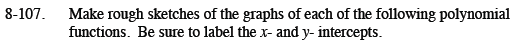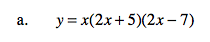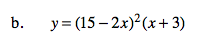Home > CCA2 > Chapter 8 > Lesson 8.2.3 > Problem8-107

8-107.
1. Make rough sketches of the graphs of each of the following polynomial functions. Be sure to label the x- and y- intercepts. Homework Help ✎

1. y = x(2x + 5)(2x − 7)

2. y = (15 − 2x)2(x + 3)Determine the x-intercepts using the Zero Product Property.

Use your graphing calculator to check your graph.This is a cubic function, but there are only two x-intercepts because on of the factors in squared. This factor creates a double root.